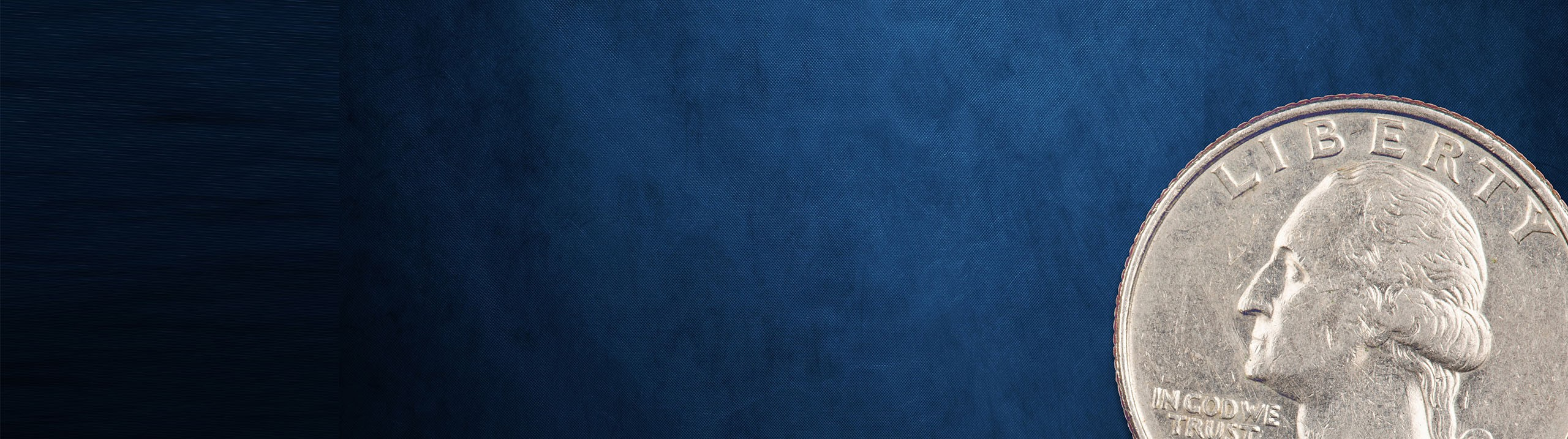## Microeconomics

Learn the toughest concepts covered in Microeconomics with step-by-step video tutorials and practice problems by world-class tutors

Perfect Competition

# Individual Supply Curve in the Short Run and Long Run

1
concept

## Individual Firm Supply Curve in the Short Run3m
Play a video:
Alright. So now that we've discussed when the firm is going to produce in the short run and the long run, let's see what the firm's supply curve is gonna look like in the short run in the long run. So, remember first, let's talk about the short run. So this is gonna be the short run first here, short run. All right. So here, in the short run, when is it that a firm does not shut down? So a firm does not shut down. It stays open when the price is greater than the average variable cost. Right? That's when they stay open. That's when they stay producing. Right. So, what we're gonna see is that the firm's short run supply curve, uh when we're dealing with perfect competition, it's gonna be the portion of the marginal cost curve above average variable cost. Okay, So that is where we produce in the short run, Right. That's how we discuss it at any price above that minimum. A VC Any of those high prices, we are going to maybe at a loss maybe at a profit, but we are going to produce at any any price above minimum. Abc. Right. So, that's what we see here on this left hand graph, right, on the left hand graph, I have all of our kurds showing. And you'll see, right, if we had a price right here, let's say this price right here, p one that I'm gonna put, we would produce, right, we would produce this quantity where the marginal revenue equals the marginal cost and anywhere, right. Any other price, I could have put a little bit higher, a little bit lower. As long as it's above that. A VC We're gonna keep producing as they cross. Right. It's gonna be all these points where marginal revenue equals marginal cost. Right? But if we were at a point below right, if we were at this price down here uh P. Two, well, we wouldn't produce, right, We're not covering our average variable cost, so we don't produce. That's why I've got that section right here. This little U shaped section of the marginal cost curve kind of shaded out, and then we've got this section right here, this red section over on the left hand side, that's a quantity of zero. Right? So any price up to that minimum abc we're gonna have a quantity of zero. So that's why we get this kind of almost a funky shape. So, I took all those cost curves out and I went over here to the right hand side and that's kind of what the supply curve looks like. So this would be the firm supply curve in the short run, right, short run. All right. So what we see is that it's at any point where the price is greater than the average variable cost, we are going to produce. Right. And that's how we have all these little points points. Points Points points points all the way up the marginal cost curve. Um And that's how our supply curve gets formed, right? So that this black dot right here, that's our minimum, a VC minimum average variable cost. Okay, A VC right there behind me. Alright, cool. So let's go ahead and discuss what the supply curve looks like in the long run. Let's do it in the next video.
2
concept

## Individual Firm Supply Curve in the Long Run3m
Play a video: